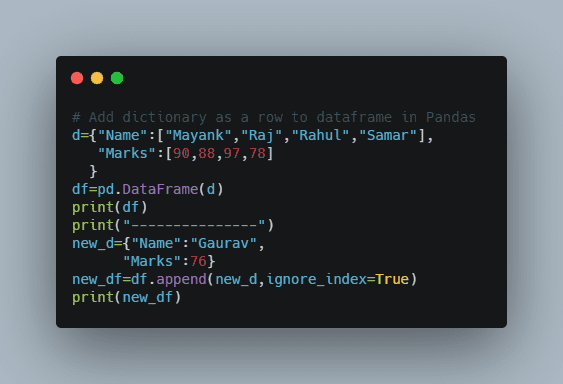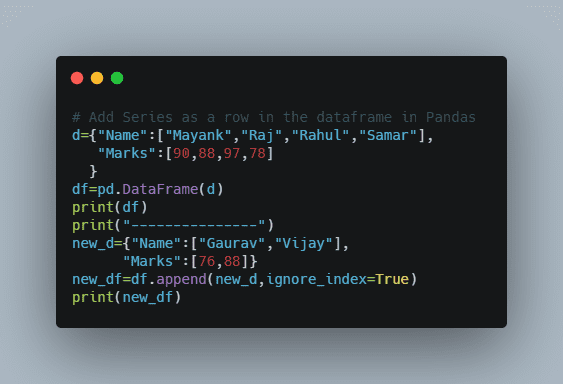# Append/Add Row to Dataframe in Pandas – dataframe.append() | How to Insert Rows to Pandas Dataframe?

Worried about how to append or add rows to a dataframe in Pandas? Then, this tutorial will guide you completely on how to append rows to a dataframe in Pandas Python using the function dataframe.append() We have listed the various methods for appending rows to a dataframe. In this tutorial, we will discuss how to append or add rows to the dataframe in Pandas. Before going to the main concept let us discuss some basic concepts about pandas and Dataframes.

## Pandas – Definition

Pandas is a package in python that is used to analyze data in a very easy way. The reason why pandas are so famous is that it is very easy to use. But we can not directly use the pandas’ package in our program. To use this package first we have to import it.

## Dataframe – Definition

Dataframe is a 2D data structure that store or represent the data in the 2D form or simply say in tabular form. The tabular form consists of rows, columns, and actual data. By using pandas we can manipulate the data as we want i.e we can see as many columns as we want or as many rows as we want. We can group the data or filter the data.

Let us understand both dataframe and pandas with an easy example

import pandas as pd
d={"Name":["Mayank","Raj","Rahul","Samar"],
"Marks":[90,88,97,78]
}
df=pd.DataFrame(d)
print(df)

Output

    Name  Marks
0  Mayank     90
1     Raj     88
2   Rahul     97
3   Samar     78

Here we see that first, we import our pandas package then we create a dictionary, and out of this dictionary, we create our dataframe. When we see our dataframe we see that it consists of rows and columns and data. There are many ways to create a dataframe like importing excel or CSV files or through a dictionary but this is not the main concern of this article.

Before understanding the concept of appending rows to a dataframe first we have to know a little bit about the append() method.

### append() method

append() method is used to append rows of other dataframe at the end of the original or given dataframe. It returns a new dataframe object. If some columns are not presented in the original dataframe but presented in a new dataframe then-new column will also be added in the dataframe and data of that column will become NAN.
Syntax: DataFrame.append(other, ignore_index=False, verify_integrity=False, sort=None)

## Ways on Pandas append row to Dataframe

### Method 1- How to Add dictionary as a row to dataframe

In this method, we see how we can append dictionaries as rows in pandas dataframe. It is a pretty simple way. We have to pass a dictionary in the append() method and our work is done. That dictionary is passed as an argument to other the parameter in the append method. Let us see this with an example.d={"Name":["Mayank","Raj","Rahul","Samar"],
"Marks":[90,88,97,78]
}
df=pd.DataFrame(d)
print(df)
print("---------------")
new_d={"Name":"Gaurav",
"Marks":76}
new_df=df.append(new_d,ignore_index=True)
print(new_df)

Output:

     Name  Marks
0  Mayank     90
1     Raj     88
2   Rahul     97
3   Samar     78
---------------
Name  Marks
0  Mayank     90
1     Raj     88
2   Rahul     97
3   Samar     78
4  Gaurav     76

Explanation:
In this example, we see how we can append a dictionary in our original dataframe. By this method, our original dataframe will not affect that why we store the new Dataframe in a new variable so that we can analyze the changes.
Instead of assigning it to a new variable, we can assign it to the original dataframe in this case our original dataframe gets modify. It means that the append() method is not inplace.
Note: Passing ignore_index=True is necessary while passing dictionary or series otherwise a TypeError error will come.

### Method 2 – Add Series as a row in the dataframe

This is another method to append rows in the dataframe. Let us see why this method is needed.d={"Name":["Mayank","Raj","Rahul","Samar"],
"Marks":[90,88,97,78]
}
df=pd.DataFrame(d)
print(df)
print("---------------")
new_d={"Name":["Gaurav","Vijay"],
"Marks":[76,88]}
new_df=df.append(new_d,ignore_index=True)
print(new_df)

Output:

    Name  Marks
0  Mayank     90
1     Raj     88
2   Rahul     97
3   Samar     78
---------------
Name     Marks
0           Mayank        90
1              Raj        88
2            Rahul        97
3            Samar        78
4  [Gaurav, Vijay]  [76, 88]

If we want to add multiple rows at one time and we try it using a dictionary then we get output like this then we get the output as shown above.

To solve this issue we use series. Let us understand what series means.

### Series

Series is a 1-D array that stores a single column or row of data in a dataframe.

syntax: pandas.Series( data, index, dtype, copy)

series=pd.Series(['Ajay','Vijay'])
print(series)
print(type(series))

Output

0     Ajay
1    Vijay
dtype: object
<class 'pandas.core.series.Series'>

That is how we can create a series in pandas. Now we see how we can append series in pandas dataframe. It is similar like as we pass our dictionary. We can simply pass series as an argument in the append() function. Let see this with an example.

d={"Name":["Mayank","Raj","Rahul","Samar"],
"Marks":[90,88,97,78]
}
df=pd.DataFrame(d)
print(df)
print("---------------")
series=[pd.Series(['Gaurav',88], index=df.columns ) ,
pd.Series(['Vijay', 99], index=df.columns )]
new_df=df.append(series,ignore_index=True)
print(new_df)

Output:

     Name  Marks
0  Mayank     90
1     Raj     88
2   Rahul     97
3   Samar     78
---------------
Name  Marks
0  Mayank     90
1     Raj     88
2   Rahul     97
3   Samar     78
4  Gaurav     88
5   Vijay     99

We see that by this method we solve the problem to add multiple rows at a time that we face in the dictionary.

### Method 3 – How to Add row from one dataframe to another dataframe

To understand this method first we have to understand about concepts of loc.

### loc[ ]

It is used to access groups of rows and columns by values. Let us understand this concept with help of an example.

students = [ ('Mayank',98) ,
('Raj', 75) ,
('Rahul', 87) ,
('Samar', 78)]
df = pd.DataFrame(  students,
columns = ['Name' , 'Marks'],
index=['a', 'b', 'c' , 'd'])
print(df)
print("------------------")
# If we want only row 'c' and all columns
print(df.loc[['c'],:])
print("------------------")
# If we want only row 'c' and only column 'Name'
print(df.loc['c']['Name'])
print("------------------")
# If we want only row 'c' and 'd' and all columns
print(df.loc[['c','d'],:])
print("------------------")
# If we want only row 'c' and 'd' and only column 'Name'
print(df.loc[['c','d'],['Name']])
print("------------------")

Output:

     Name  Marks
a  Mayank     98
b     Raj     75
c   Rahul     87
d   Samar     78
------------------
Name  Marks
c  Rahul     87
------------------
Rahul
------------------
Name  Marks
c  Rahul     87
d  Samar     78
------------------
Name
c  Rahul
d  Samar
------------------

This example is very helpful to understand how loc works in pandas.

Now it can be very easy to understand how we can add rows of one dataframe to another dataframe. Let us see this with an example.

students1 = [ ('Mayank',98) ,
('Raj', 75) ,
('Rahul', 87) ,
('Samar', 78)]
df1 = pd.DataFrame(  students,
columns = ['Name' , 'Marks'],
index=['a', 'b', 'c' , 'd'])
print(df1)
print("------------------")
students2 = [ ('Vijay',94) ,
('Sunil', 76),
('Sanjay', 80)
]
df2= pd.DataFrame(  students2,
columns = ['Name' , 'Marks'],
index=['a', 'b','c'])
print(df2)

print("------------------")
new_df=df1.append(df2.loc[['a','c'],:],ignore_index=True)
print(new_df)

Output:

     Name  Marks
a  Mayank     98
b     Raj     75
c   Rahul     87
d   Samar     78
------------------
Name  Marks
a   Vijay     94
b   Sunil     76
c  Sanjay     80
------------------
Name  Marks
0  Mayank     98
1     Raj     75
2   Rahul     87
3   Samar     78
4   Vijay     94
5  Sanjay     80

In this example, we see how we easily append rows ‘a’ and ‘c’ of df2 in df1.

### iloc[]

iloc[] in pandas allows us to retrieve a particular value belonging to a row and column using the index values assigned to it. IT will raise Index errors if a requested indexer is out-of-bounds.

students1 = [ ('Mayank',98) ,
('Raj', 75) ,
('Rahul', 87) ,
('Samar', 78)]
df1 = pd.DataFrame(  students,
columns = ['Name' , 'Marks'],
index=['a', 'b', 'c' , 'd'])
print(df1.iloc)

Output

Name     Mayank
Marks        98
Name: a, dtype: object

This example shows how we can access any row using an index.

Note: We use the index in iloc and not the column name.

Now let us see how we can append row in dataframe using iloc

students1 = [ ('Mayank',98) ,
('Raj', 75) ,
('Rahul', 87) ,
('Samar', 78)]
df1 = pd.DataFrame( students,
columns = ['Name' , 'Marks'],
index=['a', 'b', 'c' , 'd'])
print("Original dataframe")
print(df1)
print("------------------")
df1.iloc = ['Vijay', 80]
print("New dataframe")
print(df1)

Output:

Original dataframe
Name  Marks
a  Mayank     98
b     Raj     75
c   Rahul     87
d   Samar     78
------------------
New dataframe
Name  Marks
a  Mayank     98
b     Raj     75
c   Vijay     80
d   Samar     78

This example shows how we add a column in the dataframe at a specific index using iloc.

So these are the methods to add or append rows in the dataframe.

Want to expert in the python programming language? Exploring Python Data Analysis using Pandas tutorial changes your knowledge from basic to advance level in python concepts.

Read more Articles on Python Data Analysis Using Padas – Add Contents to a Dataframe